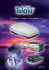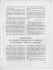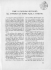# Journal of Computational Information Systems 10: 16 (2014) 6965–6972 Available at

## Transcription

Journal of Computational Information Systems 10: 16 (2014) 6965–6972 Available at
```Journal of Computational Information Systems 10: 16 (2014) 6965–6972
Available at http://www.Jofcis.com
Nonlinear Coordinated Control for SVC and Generator
Excitation Based on the IDSC Method ⋆
Yanfei ZHANG, Shaojie LU, Mingming LI, Wenlei LI ∗
School of Information Science and Engineering, Ningbo University, Ningbo 315211, China
Abstract
Based on the improve dynamic surface control (IDSC) method and disturbance attenuation technique,
the nonlinear adaptive robust controllers of power systems coordinated control for SVC and generator
excitation are proposed in this paper. The IDSC method is combined with the sliding mode control,
and the parameter adaptive laws are not based on the certainty equivalence criterion. Because during
controller design procedure besides the external disturbances are considered, some parametric uncertainties are considered as well. Thus the derived controller can guarantee the whole errors system globally
and uniformly ultimately bounded. Theory analysis and simulation results show that the proposed
coordinated controllers have the eﬀectiveness and accuracy in a single machine to inﬁnite bus (SMIB)
system.
Keywords: FACTs (Flexible AC Transmission system); SVC (Static VAR Compensator); Excitation
Control; Dynamic Surface Control
1
Introduction
The SVC is one of most widely used FACTs devices which are usually installed in long transmission
lines, and it is an eﬀective and economical means of solving problems of transient stability,
system reliability, static voltage stability, dynamic stability and steady state stability in power
regulation systems [1-3]. At the same time, the generator excitation controllers are helpful in the
enhancement of transient stability or voltage regulation. However, with only excitation control,
the system stability may not be maintained if a large fault occurs close to the generator terminal
, or the simultaneous transient stability and voltage regulation enhancement may be diﬃcult
to be achieved .
Traditionally, the generator excitation controller and SVC controller are designed separately
without considering their dynamic interconnections. So the destabilizing interactions among
controllers are possible because of the local and/or uncoordinated control strategies. In some
⋆
This work was supported by K. C. Wong Magna Fund in Ningbo University, Pivot Research Team in Scientiﬁc
and Technical Innovative of Zhejiang Province (2010R50004, 2012R10004-03).
∗
Corresponding author.
Email address: [email protected] (Wenlei LI).
DOI: 10.12733/jcis11213
Augest 15, 2014
6966
Y. Zhang et al. /Journal of Computational Information Systems 10: 16 (2014) 6965–6972
worse cases, they can even cause dynamic instability and restrict the operating power range of the
generators . For the coordination of excitation controls and FACTs controls, some approaches
have been addressed in the literature [7-9]. In those papers, the designs of controllers are based on
the approximation linearization models of a power system or the accurate mathematical models.
Whereas, due to the characteristics of nonlinearity, time variation, uncertain large disturbances
and multivariable coupling, accurate model of the power system is not available.
In this paper, the generator excitation and SVC are controlled in coordination and adjustment
at the same time by the IDSC. Comparing with the conventional DSC, the sliding mode control
 is introduced in the dynamic surface design steps, and the IDSC method can not only reduce
the computation, but also greatly improve the system robustness, speed and accuracy. As the
entire design process does not use any linear processing, we can fully use of the system nonlinearity
to ensure the applicability of proposed control law in the nonlinear systems. Simulation results
show that the proposed controllers have the eﬀectiveness and accuracy in a SMIB system.
2
System Description
The SMIB system with an SVC is shown in Fig. 1(a), and the conﬁguration of the SVC is shown
in Fig. 1(b), which consists of a ﬁxed capacitor and a thyristor controlled reactor (FC-TCR),
where BL is the inductive susceptance, BC is the capacitive susceptance, then BL + BC is the
equivalent susceptance of SVC .
*
7
E
q
XT
/
XL
XL
69&
%&
%/
D
E
Fig. 1: An SMIB power system with SVC
Supposed the mechanical input power of the generator is constant, the excitation mode is quick
response excitation, then the SVC is described by a one-order inertial loop, and the whole system
model is expressed as follows.

δ˙ = ω − ω0





D
ω0

′ VS

[P
−
(ω
−
ω
)
−
E
sin δ]
ω
˙
=

m
0
q
′

H
ω0
XdΣ

1 ′
1 Xd − Xd′
1
′
˙

E
=
−
E
+
V
cos
δ
+
u1

S
q
q
′
′


Td
Td0 XdΣ
Td0




1

 B˙ L =
(−BL + BL0 + u2 )
Tsvc
(1)
Y. Zhang et al. /Journal of Computational Information Systems 10: 16 (2014) 6965–6972
6967
where δ is the rotor angle of the generator, ω is the relative speed of the generator, Pm is
the mechanical input power of the generator, D is the damping coeﬃcient, H is the inertia
′
constant, Eq′ is the transient EMF in the quadratic axis of the generator, Td and Td0 are the time
constants of ﬁeld winding when stator winding is in open-circuit and closed-circuit, respectively,
′
Xdσ
= X1 + X2 − X1 X2 (BL + BC ) is the impedance of the whole system, X1 = Xd′ + XT + XL1 ,
X2 = XL2 , Tsvc is the time constant of SVC regulator, and u1 and u2 are the excitation control
and SVC control input, respectively.
′
= X11 − X12 (BL + BC ), where X11 = X1 + X2 , X12 = X1 × X2 , and
For system (1), deﬁne XdΣ
′
′
let x1 =δ − δ0 , x2 = ω − ω0 , x3 = Eq′ − Eq0
, x4 = BL − BL0 , where δ0 , ω0 , Eq0
and BL0 are the
initial values of corresponding variables, then system (1) is transformed into:


x˙ 1 = x2




 x˙ 2 = − D x2 + ω0 [Pm − V′S (x3 + E ′ ) × sin(x1 + δ0 )]
q0
H
H
XdΣ
(2)
′
1
1 Xd −Xd
′

x˙ 3 = − T ′ (x3 + Eq0 ) + Td0 X ′ VS × cos(x1 + δ0 ) + T1d0 u1


d
dΣ


 x˙ = 1 (−x + u )
4
We set k1 =
D,
Td′
ω0
,
H
k2 =
Tsvc
ω0 VS
,
′
XdΣ
k3 =
4
2
(Xd −Xd′ )VS
Td0
are unknown parameter, then θ2 =
and assume they are known constants, and supposed
D
−H
and θ3 = − T1′ are also unknown parameters.
d
Based on above deﬁnitions, system (2) is rewritten as the following:
x˙ 1 = x2
(3a)
′
x˙ 2 = θ2 x2 + k1 Pm − k2 (x3 + Eq0
) × sin(δ0 + x1 ) + d1
(3b)
′
x˙ 3 = θ3 (x3 + Eq0
)+
(3c)
x˙ 4 =
3
k3 cos(x1 +δ0 )
X11 −X12 (x4 +BL0 +BC )
+
1
u
Td0 1
+ d2
1
(−x4 + u2 ) + d3
Tsvc
(3d)
Design of Nonlinear IDSC Robust Controller
For system (3), we ﬁrst deﬁne the surface error as follows.
ei = xi − xid
(4)
where x1d is the reference trajectory, xid (i = 2, 3) will be given later on by the ﬁrst order ﬁlter.
Deﬁne the boundary layer errors as
yi+1 = x(i+1)d − x∗i+1
(5)
where x∗i+1 (i = 1, 2) is the stabilizing function which will also be designed later on.
Now we shall show a new dynamic surface control procedure of the robust adaptive controller
for the system deﬁned in (3).
Step1: For the ﬁrst subsystem of (3), viewing x2 as the virtual control, we have
e˙ 1 = x˙ 1 − x˙ 1d = x2 x˙ 1d = e2 + y2 + x∗2 − x˙ 1d
(6)
6968
Y. Zhang et al. /Journal of Computational Information Systems 10: 16 (2014) 6965–6972
We select the ﬁrst virtual stabilizing function
x∗2 = −c1 e1 + x˙ 1d
(7)
where c1, γ 1 are the positive design constants. Substituting (7) into (6) it yields
e˙ 1 = −c1 e1 + e2 + y2
(8)
Let x∗2 be an input and passed through a ﬁrst-order ﬁlter as follows,
τ2 x˙ 2d + x2d = x∗2
(9)
where τ 2 is a given time constant, and x2d (0) = x∗2 (0).
Step 2: For the second subsystem of (3), viewing x3 as the virtual control, we have
′
e˙ 2 = x˙ 2 − x˙ 2d = θ2 x2 + k1 Pm − k2 (x3 + Eq0
) × sin(δ0 + x1 ) + d1 − x˙ 2d
1
′
= k1 Pm − k2 sin(δ0 + x1 ) × [(e3 + y3 + x3∗ + Eq0
)] + x2 (θˆ2 + x22 − z2 ) + d1 − x˙ 2d
2
(10)
where θˆ2 is the parameter estimation of θ2 , and z2 is the parameter estimation errors and given
by
z2 = θˆ2 − θ2 + x22 /2
(11)
Select the second virtual stabilizing function
x∗3 =
1
[−c2 e2
k2 sin(δ0 +x1 )
− k1 Pm − x2 × (θˆ2 + 12 x22 ) −
e2
(2γ2 )2
′
+ x˙ 2d ] − Eq0
(12)
where c2, γ 2 are positive design constants, and the adaptive law to the uncertain parameter is
selected as
˙
′
(13)
θˆ2 = −x2 [k1 Pm − k2 sin(δ0 + x1 ) × (x3 + Eq0
) + x2 (θˆ2 + 12 x22 )]
Therefore the dynamics of the estimation errors is
z˙2 = −x22 z2 + x2 d1
(14)
Substituting (11) and (12) into (10) yields
e˙ 2 = −c2 e2 −
e2
(2γ2 )2
− x2 z2 + k2 sin(δ0 + x1 )(e3 + y3 ) + d1
(15)
Let x∗3 be an input and passed through a ﬁrst-order ﬁlter as follows.
τ3 x˙ 3d + x3d = x∗3
(16)
where τ3 is a given time constant, and x3d (0) = x∗3 (0)
Step 3: For the third subsystem of (3), in light of (4), we have
1
k3 cos(x1 + δ0 )
+
u1 + d2 − x˙ 3d
X11 − X12 (x4 + BL0 + BC ) Td0
1
1
k3 cos(x1 + δ0 )
′
= (x3 + Eq0
)(θˆ3 + x23 − z3 ) +
u1 + d2 − x˙ 3d +
2
Td0
X11 − X12 (e4 + y4 + x∗4 + BL0 + BC )
(17)
′
e˙ 3 = x˙ 3 − x˙ 3d = θ3 (x3 + Eq0
)+
Y. Zhang et al. /Journal of Computational Information Systems 10: 16 (2014) 6965–6972
6969
where θˆ3 is the parameter estimation of θ3 , and z3 is the parameter estimation errors and given
by
z3 = θˆ3 − θ3 + x23 /2
(18)
Select the third virtual stabilizing function
x∗4 =
X11 k3 cos(x1 + δ0 )
+
− BL0 − BC
X12
X12 c31 e3
(19)
and excitation control u1 as
1
e3
′
u1 = Td0 [−c32 e3 − (x3 + Eq0
)(θˆ3 + x23 ) −
+ x˙ 3d ]
2
(2γ3 )2
(20)
where c31 , c32 , γ3 are positive design constants, and the adaptive law to the uncertain parameter
is selected as
1
˙
θˆ3 = −x23 (θˆ3 + x23 )
(21)
2
Therefore the dynamics of the estimation errors is
z˙3 = −x32 z3 + x3 d2
(22)
Substituting (18), (19) and (20) into (17) yields
e˙ 3 = −c3 e3 −
e3
k3 cos(x1 + δ0 )
−
x
z
+
+ d2
3
3
(2γ3 )2
X11 − X12 (e4 + y4 )
(23)
where c3 = c31 + c32 .
Let x∗4 be an input and passed through a ﬁrst-order ﬁlter as follows,
τ4 x˙ 4d + x4d = x∗4
(24)
where τ4 is a given time constant, and x4d (0) = x∗4 (0).
Remark 1. The virtual controls (19) together with excitation control (20) stabilize the third
system dynamic surface. For excitation control (20), besides it can stabilize the system dynamics,
it also controls the uncertain parameter adaptation.
Step 4: Deﬁne the sliding mode s = b1 e1 + b2 e2 + b3 e3 + e4 = 0 which satisﬁes asymptotic
reached condition, where b1 to b3 are the positive design constants, and deﬁne the Lyapunov
function of whole system as follow.
1∑ 2 1∑ 2 1 2 ε∑ 2
e +
y + s +
z
2 i=1 i 2 i=2 i 2
2 i=1 i
3
V =
4
2
(25)
where ε>0 is a design constant. The time derivative of V is
V˙ =
3
∑
i=1
ei e˙ i +
4
∑
i=2
yi y˙i + ss˙ + ε
2
∑
i=1
zi z˙i
(26)
6970
Y. Zhang et al. /Journal of Computational Information Systems 10: 16 (2014) 6965–6972
Noting that (10) and x˙ (i+1)d =
x∗i+1 −x(i+1)d
,
τi+1
y˙ i+1 = x˙ (i+1)d − x˙ ∗i+1 =
we can get
x∗i+1 − x(i+1)d
−yi+1
− x˙ ∗i+1 =
− x˙ ∗i+1
τi+1
τi+1
Let Bi+1 = x˙ ∗i+1 , and assume sup|Bi | = Di , then we have
e2
V˙ = e1 (−c1 e1 + e2 + y2 ) + e2 [−c2 e2 −
− x2 z + k2 sin(δ0 + x1 )(e3 + y3 ) + d1 ]
(2γ2 )2
4
∑
e3
k3 cos(x1 + δ0 )
−yi
+ e3 [−c3 e3 −
− x 3 z3 +
+ d2 ] +
yi (
+ Di ) + s[b1 e˙ 1
2
(2γ3 )
X11 − X12 (e4 + y4 )
τi
i=2
+ b2 e˙ 2 + b3 e˙ 3 +
(27)
(28)
1
(−x4 + u2 ) + d3 − x˙ 4d ] − εx22 z23 + εx2 z2 d1 − εx23 z32 + εx3 z3 d2
Tsvc
Select the real SVC control input u2 as
u2 = x4 + Tsvc [−β1 s − β2 sgn(s) − b1 e˙ 1 − b2 e˙ 2 − b3 e˙ 3 −
s
]
+ x˙ 4d
(2γ4 )2
(29)
where β 1 , β 2 , γ 4 are the positive design constants. Then we have
e˙ 4 = −β1 s − β2 sgn(s) − b1 e˙ 1 − b2 e˙ 2 − b3 e˙ 3 −
s
+ d3
(2γ4 )2
(30)
In the new coordinate deﬁned by (6)-(30), we have an important theorem as follows.
Theorem 1 For nonlinear systems (3) in parameter feedback form with parameter uncertainty
and external disturbances, the closed-loop errors system will be globally and uniformly ultimately
bounded if we apply the robust adaptive control law (20), (29), the stabilizing function (7), (12),
(19) and the parameter adaptive laws (13), (21). Furthermore, Given any constant µ∗ , there
exists T such that e(t)≤µ∗ for all t ≥ T .
Proof Omitted.
4
Simulation Results
According to the design results of foregoing section, we simulate closed-loop system. The simulation parameters are taken as follows: b1 =0.3, b2 =0.05, b3 =0.05, b4 =2, β1 =80, β2 =2, c1 =100,
c2 =30, c2 =30, ε=4, σ=10, γ=0.2, τ2 = τ3 = τ4 =0.01.
′
The used system parameters are in the following: H=8, Eq =1, Vs =1, X1 =0.6, X2 =0.4, Xd =1.
′
863, Xd =0.657, XT =0.127, XL1 =0.9053, XL2 =0.2426, X11 =1.6893, BL0 = BC0 =0.1587, Td0 =0.0
5, δ0 =0.23π, ω0 =100π rad/s. The simulation result is shown in Fig. 2.
The fault considered is a symmetrical three-phase short circuit fault which occurs on one of the
transmission lines at t = 0.1s, and it is removed at t = 0.25s.
Fig. 2 to Fig. 4 show the system responses as a result of the coordinated excitation and SVC
scheme. It can be seen that, compared with single excitation control, coordinated control preserves
rotor angle and relative speed stability of the synchronous generator, achieves terminal voltage
regulation and provides satisfactory system damping. This is practically desirable criterion.
Y. Zhang et al. /Journal of Computational Information Systems 10: 16 (2014) 6965–6972
Fig. 2: Rotor angle responding curves of the system
Fig. 3: Relative speed responding curves of the system
Fig. 4: Terminal Voltage responding curves of the system
6971
6972
5
Y. Zhang et al. /Journal of Computational Information Systems 10: 16 (2014) 6965–6972
Conclusions
A nonlinear adaptive coordinated control for SVC and generator excitation is designed using the
IDSC combined with the sliding mode control. This method not only reduced the computation,
but also greatly improved the system robustness and performance. Owing to the internal and
external disturbances in controller design are considered together, so the controller can not only
guarantee the states is bounded, but also attenuate the eﬀects of disturbances on states. In
internal disturbance the damping coeﬃcient uncertainty is considered, thus the controller is robust
to the system parameter variety also. The further simulations demonstrated the eﬀectiveness of
controller.
References

R. L. Hauth, T. Humann, and R. J.Newell. Application of a Static VAR system to regulate system
voltage in western Nebraska, IEEE Trans on Power Apparatus and Systems, 97 (1978) 1955-1964.

A. Luo, Z. Shuai, W. Zhu, Z. J. Shen, Combined system for harmonic suppression and reactive
power compensation, IEEE Tran. on Industry Electronics, 56 (2009) 418-428.

E. Zhijun, D. Z. Fang, K. W. Chan, S. Q. Yuan, Hybrid simulation of power systems with SVC
dynamic phasor model, International Journal of Electrical Power and Energy Systems, 31 (2009)
175-180.

E. Z. Zhou, Application of Static Var Compensators to increase power system damping, IEEE
Trans Power Syst., (8) 1993 655-661.

K. T. Law, D. J. Hill, N. R. Godfrey, Robust controller structure for coordinated power system
voltage regulator and stabilizer design, IEEE Trans Control Syst Technol., (2) 1994 220-232.

X. Lei, E. N. Lerch, D. Povh, Optimization and coordination of damping controls for improving
system dynamic performance, IEEE Trans Power Syst., (16) 2001 473-480.

A. H. Rahim, and S. G. Nassimi, Synchronous generator damping enhancement through coordinated control of exciter and AVC, IEE Proc.Part C, Gener. Trans. and Distrib., (143) 1996
211-218.

A. R. Mehran, B. W. Hogg, and M. L. El-sayed, Coordinated control of synchronous generator
excitation and static var compensator, IEEE. Trans. Energy Conv., (7) 1992 615-622.

Y. Y. Wang, Y. L. Tan, Robust Nonlinear coordinated generator excitation and svc control for
power systems, International Journal of ElectricalPower and Energy Systems, (22) 2000 187-195.
 Z. Q. Wu, Y. C. Tang, Fuzzy adaptive backstepping sliding mode control for the hydraulic gap
nonlinear system, Journal of Computational Information Systems, 9 (2013) 1113-1120.
 J. Lu, M. M. Li, W. L. Li, Nonlinear Robust control for static var compensator based on improved
backstepping method, Journal of Computational Information Systems, 10 (2014) 341-347.
```

### UNITROL Control Upgrade Extend your system's life time with minimal cost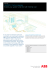### gas generator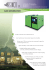### UNI World Executive Board 2015 - 2018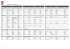### 11\ ~CAWLI\1 t - Florida Public Service Commission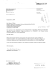### directory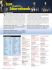### Lullaby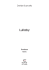### steam generator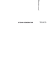### Case Study - Wegz Stadium Bar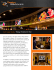### 25°C - Vanmeenen